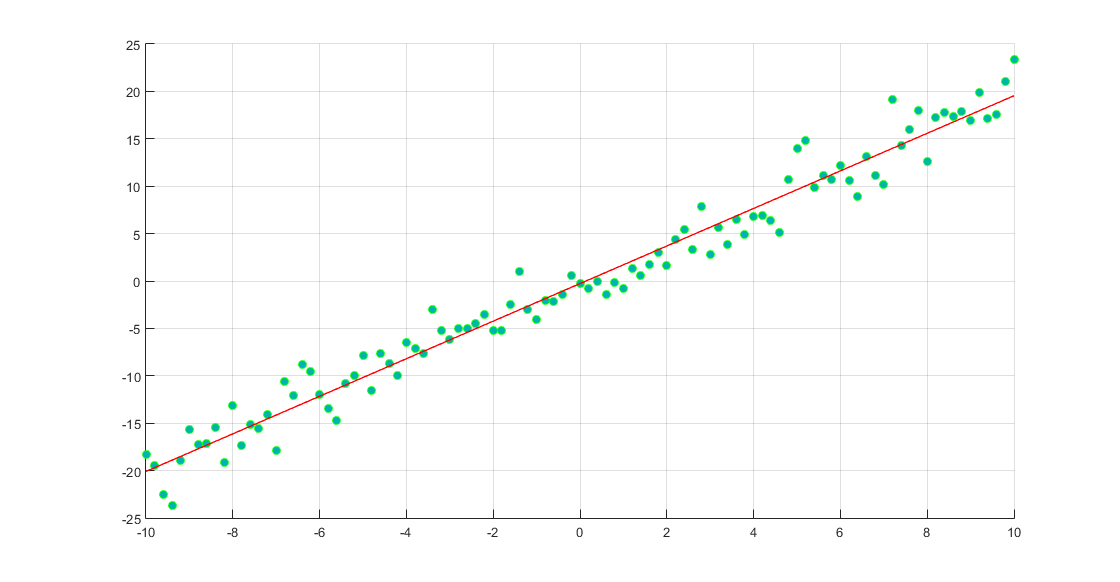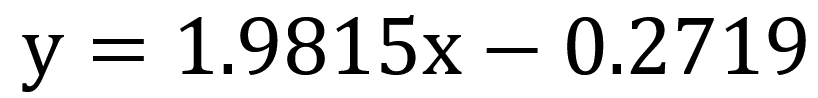#0
0
01. 云栖社区>
2. 博客>
3. 正文

## 机器学习之线性回归的最小二乘法求解

``````t=randn(1,101);

x=[-10:0.2:10];
y=2*x+t*2;
s=scatter(x,y);
s.LineWidth = 0.6;
s.MarkerEdgeColor = 'g';
s.MarkerFaceColor = [0 0.7 0.7];
hold on;

p=polyfit(x,y,1)
y1=polyval(p,x);
plot(x,y1,'-r','LineWidth',1);

grid on;  ````````````p =

1.9815   -0.2719``````+ 关注